# Using ListConvolve for the Explicite Finite Difference Method

### Developing an explicite finite difference method for the Black-Scholes equation

The partial differential equation from Black-Scholes for a derivate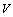on the securityis given as

``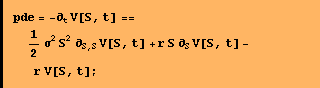``

Numerical approximation for the partial derivates follow for example from the Taylor series. The parital derivates in the equation above are replaced through the appropriate Taylor series

``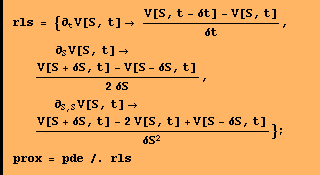``
``

In the next step the notation is changed to make it more consistent with a grid scheme.

````
`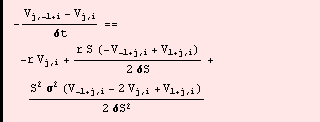`

To better illustrate the structure of the equation some more notational adjustments are made. The new structur will later help to simplify the calculations

``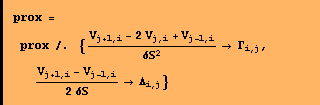``
`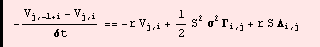`

Solving the last expression for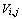and simplifying leads to

``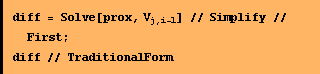``
`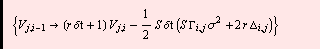`

The last expression can be rewritten as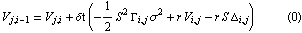withand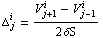.
The presented calculation scheme is a version of the explicit finite difference method. While applying this calculation schemebei the new values for the derivateare stepwise calculated fromand. Picture 1 illustrates this approach.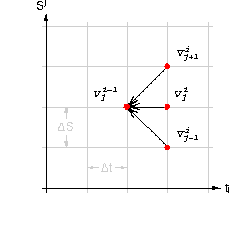### Implementing the numerical method with ListConvolve

An efficient Mathematica function for the calculation of the differences needed in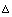andis available through ListConvolve. To demonstrate this  ListConvolve is applied to a list of symbols.

````
`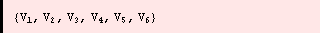`

ListConvolve used for  Δ  results in the following expression

````
``

The first list in ListConvolve, the kernel {1,0,1}, is applied piecewise to the second list and multiplicates the elements the elements of the second list and adds them up according to the values given in the kernel. This operation runs internally in Mathematica and is much faster as any loop written in Mathematica code.

The approach used for Δ can also be applied for the calculation of  Γ. ListConvolve can therefore replace loops which are common to the explicit approximation of partial differential equations.

````
``

To complete the specification of the finite difference method for the numerical solution of partial differential equations boundary and initial conditions are needed. These result from the given valuation problem.

### Example: Numerical valuation of a call option

To illustrate the application of the method the following call option will be valued

````

The number of grid points for the discrete prices of the stockcan freely be chosen within a specific range. Increasing the number of time steps improves the accuracy but also increases the overall calculation time. For a first demonstration the number of discrete stock prices is set to 20.

````

The grid points for the stock price should be placed in a range not to tight around the current stock price.  In this example the reange is choosen from zero  up to twice the strike price. From the chosen region results the step size ΔS for the discretization of the stock prices range. One way to generate the list of grid points is to use NestList .  #+ΔS& within NestList is a generic function defined for local use.
On the list of discrete stock prices the exercise function of the option can be applied. The resulting list provides the starting or initial values for the numerical method.

````

The necessary number of time steps for the explicite FDM to converge depends on the step size for the discretization of the stock price, the volatility and the strike price (see Willmot , p. 629). Using the formula given in  Willmot  the number of time steps can be calculated as

``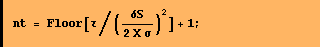``

Then the size of the time steps are

````

For the calculation of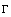andthe Mathematica function ListConvolve is used as explained above. (Attention: Before executing the following Mathematica code make sure that V is initiated with numbers.)

``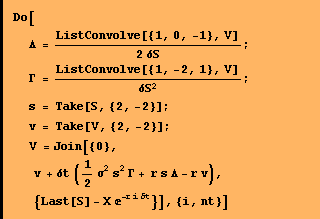``

After the loop is finished V contains a list of option values. Each option value corresponds to a discrete stock price on the grid. Interplation on these numbers can be used to calculate the option price for the current price of the underlying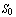.

``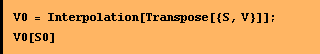``
`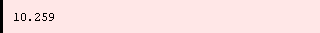`

### References

Clewlow, L./Strickland, C., 1998, Implementing Derivatives Models, John Wiley & Sons Ltd.

Willmot, P., 1998,  Derivatives: The theory and practice of financial engineering, John Wiley & Sons Ltd.

Converted by Mathematica      October 5, 2000